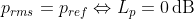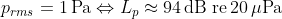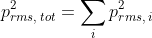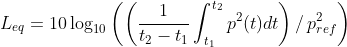This document points out a set of basic acoustic formulas, also used inside the Dewesoft Sound Level Meter SW application, in order to help users with similar calculations for customized scenarios, where math modules, formulas and C++ scripts are included.

Sound Pressure Levels

The human auditory system can understand sound pressure variations over a range of more than a million times. Due to this wide range, acoustic quantities are normally measured on a logarithmic scale with the unit decibel [dB]. The results are referred to as levels

The rms Sound Pressure Level (SPL) is defined as:where p_ref is the reference sound pressure.

The reference sound pressure is 20 μPa for sound waves in air, corresponding to around the lowest audible sound at 1 kHz for humans.

This means for example that:

• if the measured sound pressure is equal to the reference sound presure, then the (SPL) level is 0 dB:• if the measured sound pressure is 1 Pa, then the level is approximately 94 dB:• if the measured sound pressure is 0.5 Pa, then the level is approximately 88 dB:Hereby it can be observed that when the sound pressure changes with a factor of 2 then the SPL changes 6 dB.

For sound in other fluids than atmospheric air, like water, the reference sound pressure is 1 μPa. To avoid misunderstandings the reference sound pressure is sometimes stated explicitly after the level, e.g. 'XX dB re 20 μPa'.

When several independent sources of noise or sound are present at the same time, then the mean square sound pressures can be added together:Note that hereby, the total SPL is not determined by adding together multiple SPLs, but by adding together mean square sound pressures, and then calculating the total SPL:If you already have multiple SPLs that you want to add together, then the formula for the total SPL can be rewritten to use the individual SPLs instead of using the individual sound pressures, like:The formulas above can also be use to e.g. add together individual fractional octave bands.

Substracting a level from another level

Like adding mean square sound pressures, one can also do corrections to sound pressure measurements, by e.g. substracting steady background noise, or other undesirable sound pressures, from the total SPL.

For example, by measuring the total SPL and the background SPL, one can determine the SPL from the present sound sources by:Equivalent sound pressure levels

The equivalent sound pressure level is the rms sound pressure level (SPL) determined with a specified integration time:If the noise level changes regularly, e.g. by having a machine that operates at different modes in different time periods, then the equivalent sound pressure level can be calculated by:In this way the mean square sound pressures are added together with a weighting that relates to the time fraction of each noise level. T is the total measurement time.

Sound Exposure Levels

The sound exposure level, also referred to as SEL, is a quantity used for measuring the total energy of a noise event like an explosion or an aircraft take-off, independently of the duration of the event.

The sound exposure level is closely related to the equivalent sound pressure level, but instead of dividing the time integral by the actual integration time, one instead divides by t_0 = 1 s. Hereby, the sound exposure level is a measure of the total noise energy, normalized to 1 s:The measurement interval must include the full noise event.

References

• Fundamentals of Acoustics and Noise Control, by Finn Jacobsen, Torben Poulsen, Jens Holger Rindel, Anders Christian Gade and Mogens Ohlrich, DTU Denmark, dept. of Electrical Engineering.